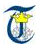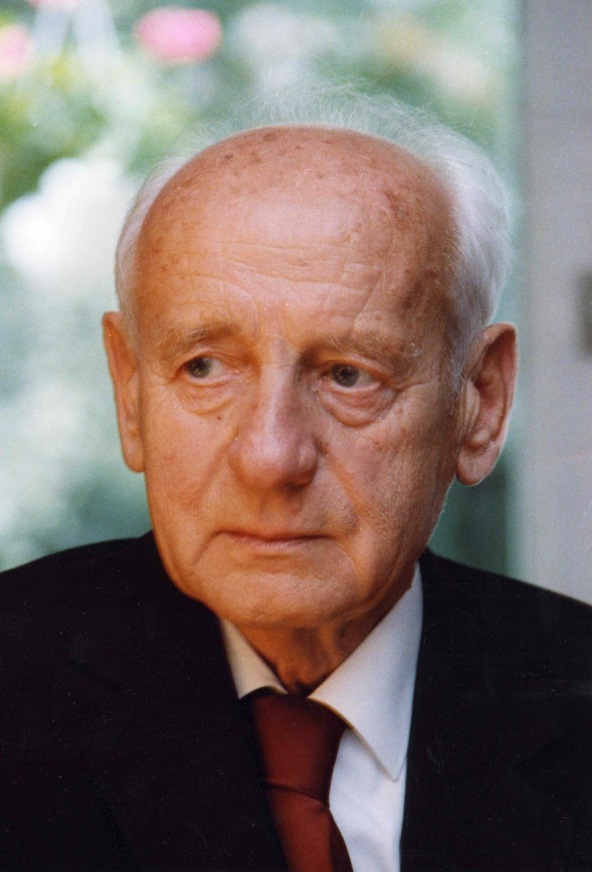Alterman Conference on Geometric Algebra  and Summer School on Kähler CalculusENID & STANLEY ALTERMAN August 1st- 9th 2016             Brasov (Romania)   Summer School Teachers First Part: Rafal Ablamowicz, Zbigniew Oziewicz, Jose Vargas, Nikolay Marchuk, Ramon González. Second and Third Parts: Jose Vargas, Zbigniew Oziewicz   Timetable The timetable of the Summer School can be downloaded here.   Program  (updated on April, 6th) First Part: Introduction to Geometric Algebra (1st to 3rd August) Introduction. Two pedestrian approaches to exterior algebra. Two pedestrian approaches to Clifford algebra. Comparison of exterior and Clifford algebras, grading and the common module. Basic examples of Clifford algebra. Development of Clifford concepts: using Pauli (3-D) algebra. Grade involution, reversion and Clifford conjugation. The even subalgebra. The unit pseudo-scalar. Hodge dual of a Clifford element. Reciprocal frames. Vector product. Reflections and rotations. Product of rotations. Lorentz transformations. Revisited concepts, now in the Dirac algebra (4-D). Lorentz transformations. We are not overlooking: complex algebra and quaternion algebra. Arbitrary dimension. Exterior products: linear independence, volumes, exterior products of multivectors. Clifford product of vector by multivector. Grade involution. Product of a vector by an arbitrary element of the algebra. Exchange of the orders of factors. Distributive property of the interior product of a vector with respect to exterior and Clifford products. Interior products of multivectors. Connection of pedestrian approaches with formal definitions. Idempotents. Ideals. Decompositions. Application of geometric algebra to plane geometry. The Euclidean and hyperbolic planes. Complex versus hyperbolic analysis. Euclidean and hyperbolic reflections and rotations, and other transformations of vectors. Similarity of triangles expressed with geometric algebra. Notable points of a triangle. Euler's line and its direction vector. Proofs of theorems of plane geometry with geometric algebra. Projective cross ratio and a proof of Chasles' theorem. Application of geometric algebra to the three-dimensional spaces. Hamilton's definition of quaternions as quotients of vectors in the Euclidean space. Rotations and its composition. Barycenter, circumcentre, incentre, Monge's point and Euler's line of a tetrahedron. Spherical trigonometry. Lobachevsky geometry and trigonometry in the pseudoEuclidean space.  Tutorial on CLIFFORD with eClifford and Bigebra: A Maple Package for Clifford and Grassmann Algebras. A Maple Package for Clifford and Grassmann Algebras. A quick start on Clp,q(B) or Clp,q,r  in CLIFFORD or eCLIFFORD. Notation and basic computations in more details. Built-in database in Clifford algebras Clp,q when p+q ≤ 9. Mathematical design of CLIFFORD based on Chevalley's definition of Clifford algebra. Algorithms for Clifford product in Cl(B): cmulNUM, cmulRS, cmulWalsh3 for algebras Clp,q. Special new package eCLIFFORD for fast computations in Clifford algebras Clp,q,r. A fast algorithm ecmul for Clifford product in Clp,q,r based on Walsh functions (a modified cmulWalsh3). Algebraic operations in Cl(B). Research with CLIFFORD and related packages, such as SymGroupAlgebra. On parallelizing the Clifford product in CLIFFORD. Using periodicity theorems in higher dimensional Clifford algebras or using eCLIFFORD. Matrix representations and spinors. Representation of Clifford algebras with square matrices. Table of matrix representations. Isomorphisms of Clifford algebras. Periodicity theorems. Spinors. Application of spinors to quantum mechanics. Exterior Calculus. The concept of differential form in Kähler. Revisiting concepts of exterior algebra. The exterior derivative and general Stokes theorem. Maxwell's equations. Currents as Cartan invariants not at constant time. The true nature of the energy-momentum tensor.   Second Part: Kähler calculus (4th to 6th August) August 4th Concept of Kähler algebra and of its differential forms. The Kähler algebra of the real plane and its even subalgebra. The angular integral. Splits and subsplits of spacetime's Kähler algebra. Idempotents. Ideals. Primitive idempotents. Theorems of residues and of Cauchy by means of just real algebra and standard calculus. Templates of ideals to come down from 32 real components of "wave functions" to 16 and then 8 components (Dirac spinors) in complex spacetime Kähler algebra. August 5th Kähler on the general differentiaiton of (p,q)-tensor-valued differential r-forms. The Kähler and Cartan approaches to Kähler differentiation. Covariant differentiation. Leibniz rules. Hodge dual, co-derivative and Laplacians. Harmonic, strict harmonic and constant differentials. Helmholtz theorem for differential 1-forms and 2-forms in 3-D Euclidean space. Cauchy calculus with real differential forms. Cauchy calculus versus exterior and Kähler calculus. August 6th Solutions with time symmetry of exterior systems. Kähler equations. Electromagnetic coupling. Dominant energy solutions. Kähler version of the Pauli-Dirac equation and of the Foldy-Wouthuysen Hamiltonian and comparison with standard relativistic quantum mechanics. The fine structure of the hydrogen atom. Dirac theory as contrived, non-spontaneous, when judged from Kähler's theory.   Third part: Applications of Kähler Calculus (8th to 9th August) August 8th Lie derivatives as partial derivatives. Separation of the emerging spin and orbital terms of the third component of total angular momentum. Killing symmetry. The algebraic form of the spin operator. Total angular momentum and its square. Scalar products of differential forms of different grades. A quadratic conservation law in the Kähler calculus. The emergence of the concept of charge. Superseding of Hodge's theorem by solving the exterior system that specifies the curl and divergence of a differential form of homogeneous grade. Further showing of Dirac theory as contrived, non-spontaneous, when judged from Kähler's theory. August 9th Rotations with Clifford algebra. Geometrization of the imaginary unit in relativistic quantum mechanics. Real idempotents for rotational and time translation symmetries. Tensor product of Kähler algebra and tangent Clifford algebra. Reflection on the foundations of differential geometry as argument for canonical Kaluza-Klein geometry. Impact on our understanding of flat spacetime geometry. Extension of positron-electron to other leptonic generations. Mirror symmetric elements of that tensor product of algebras. Implications of the commutativity of those elements for the extension of the system of primitive idempotents. Quarks.    FACULTY OF MATHEMATICS & INFORMATICSTRANSILVANIA UNIVERSITY OF BRASOVERICH KÄHLER Dedication Welcome Introduction Committees The Event Venue Topics Schedule Summer School Conference About Kähler Biographical note Kähler calculus Notes on K. C. Participation You count Fees Registration Submission Q and A Logistics Food Lodging Transport "Tourist" information Sponsors Contact